# Christoffel Symbol

## Christoffel Symbol

The Christoffel symbol of a quadratic differential form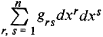is a symbol for the abbreviated representation of the expression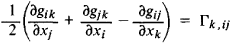The symbol Γk, ij is called the Christoffel symbol of the first kind in contrast to the Christoffel symbol of the second kind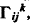which is defined by the relation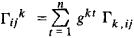where gkt is determined from the equalities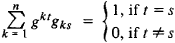The symbols were introduced by E. Christofl’el in 1869.

References in periodicals archive ?
The non-vanishing independent components of the christoffel symbol [MATHEMATICAL EXPRESSION NOT REPRODUCIBLE IN ASCII.]
As an example, in the construction of GR, the role of Christoffel symbol is obvious in describing both field and motion.
where [[GAMMA].sup.[rho].sub.[mu][nu]] is the Christoffel symbols second kind and the square brackets mean
Following the cited book we call these functions the Christoffel symbols of [nabla] with respect to the chart h and the local frame field [S.sub.U].
Concretely, the metric tensor, the determinant of metric matrix field, the Christoffel symbols, and Riemann tensors on the 3D domain are expressed by those on the 2D surface, which are featured by the asymptotic expressions with respect to the variable in the direction of thickness of the shell.
where the Christoffel symbols [[GAMMA].sup.[lambda].sub.[mu]v] are
The components of the corresponding metric tensor h and the Christoffel symbols on the manifold N will be denoted by [h.sub.[alpha][beta]],[H.sup.[alpha].sub.[beta][gamma]].
Now, an alternative (although implicit) definition of the Christoffel symbols is contained in the equation that states the vanishing of covariant derivatives of the metric:
This theory has some advantages over the general relativity; the quantities such as christoffel symbols and others become tensors which otherwise in Riemannian geometry they are not.
One straightforwardly goes through the tedious calculation of the Christoffel symbols and the components of the Ricci tensor, finding:
If (U, [x.sup.1], ..., [x.sup.n]) is a coordinate chart on M, then the Christoffel symbols [[GAMMA].sup.k.sub.ij] of the Levi-Civita connection are related to the functions [g.sub.ij] by the formulas

Site: Follow: Share:
Open / Close